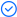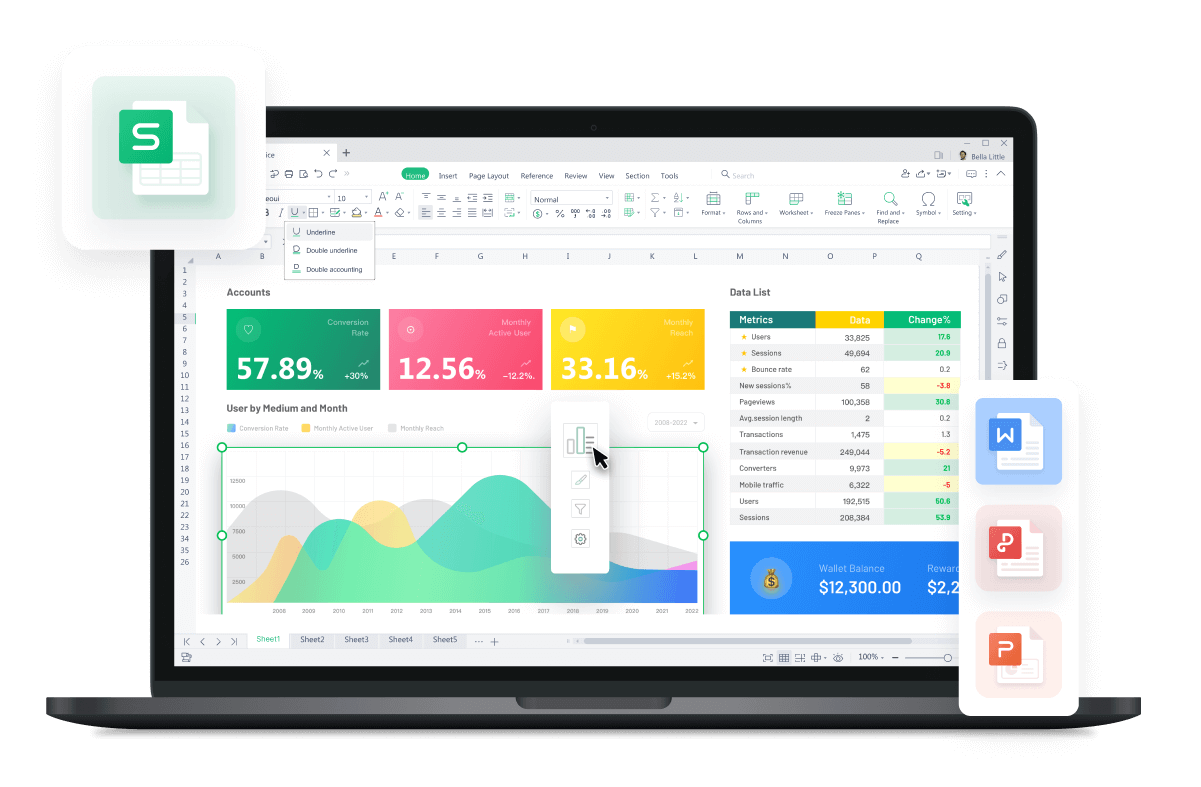WPS Office

Free All-in-One Office Suite with PDF EditorRead, edit, and convert PDFs with the powerful PDF toolkit.Microsoft-like interface, easy to use.

Windows • MacOS • Linux • iOS • Android# How to Add Text After Formula In Excel （Easy Steps）

August 1, 2022
4.0K Views

Excel has been used as a tool to help in organizing and analyzing the data. However, often times we would require to use couple of Formulas to write in the Excel to perform different functions, required at that time. As the default nature of Excel contains only the formula values in the Excel. Therefore, most of the times it is difficult to understand the complexity of the formula and the overall analysis of the situation. However, by the use of addition of several different lines in the formula can prove to be an important step to all of the individuals reading the excel cell. Therefore, this article will help you in adding Formula and Text in the same Excel Cell using 3 different Functions.

Add Text and Formula in the Same Cell in Excel

Following database contains crucial values of different aspects of the individual’s salary. However, in the dataset, it is clearly not understandable of what actually is data representing. Therefore, we would be requiring to add some text in order to make it more presentable to the audience that is reading this sheet.

1. First step is to Enter the Database in the Excel Cell.

2. Type in the formula to your designated cell in order to perform the function.

=A2 & “’s monthly savings is:” &B2-C2

Whereas, the B2, C2, and A2 are the Cell Names this dataset is containing the information.

3. You will see the result as:

4. To use and apply the same formula to different cells in the excel, use the fill handle to make sure that all cells have desired value.

Congratulations, you have successfully used the & function in order to use text and formula in the same Excel cell.

Add Text and Formula in the Same Cell Using Text Function

Following database consists of the workhours that will be calculated in order to pay to the employees. In the dataset that has been provided below, it will take a lot time to calculate when it comes to this type of cell arrangement in the excel. Therefore, using the Text function in the Excel cell will help to make the Work Hours in a separate column.

1. First step is to Enter the Database in the excel cell.

2. Type in the formula to your designated cell in order to perform the function.

=A17 & “’s total work hours are: “& TEXT (C17-B17, “h”)

Whereas, the A17, B17, and C17 are the Cell Names this dataset is containing the information.

3. You will see the result as:

4. To use and apply the same formula to different cells in the excel, use the fill handle to make sure that all cells have desired value.

Congratulations, you have successfully used the TEXT function in order to use text and formula in the same Excel cell

At Any Sequence- Add Text After Formula

Using the text and the formula at any sequence in the same cell.

1. First step is to Enter the Database in the excel cell.

2. Type in the formula to your designated cell in order to perform the function.

=Monthly saving of Robert is: &C5-D5

Whereas, the B5, C5, and D5 are the Cell Names this dataset is containing the information.

3. You will see the result as:

4. To use and apply the same formula to different cells in the excel, use the fill handle to make sure that all cells have desired value.